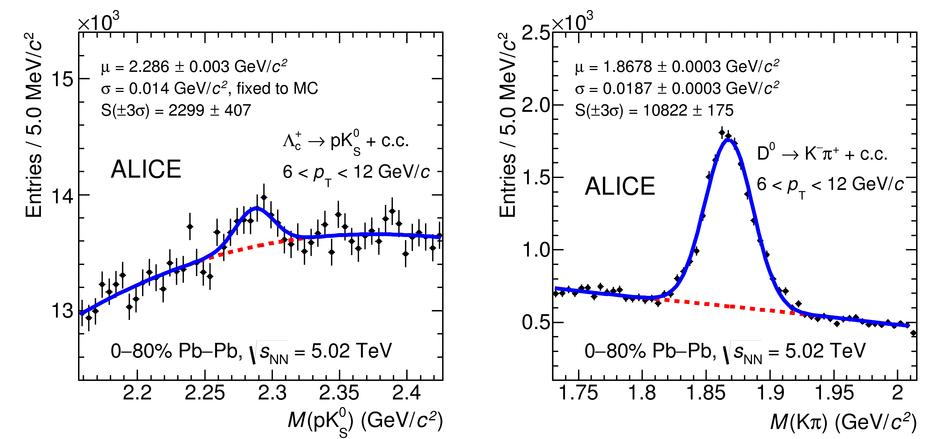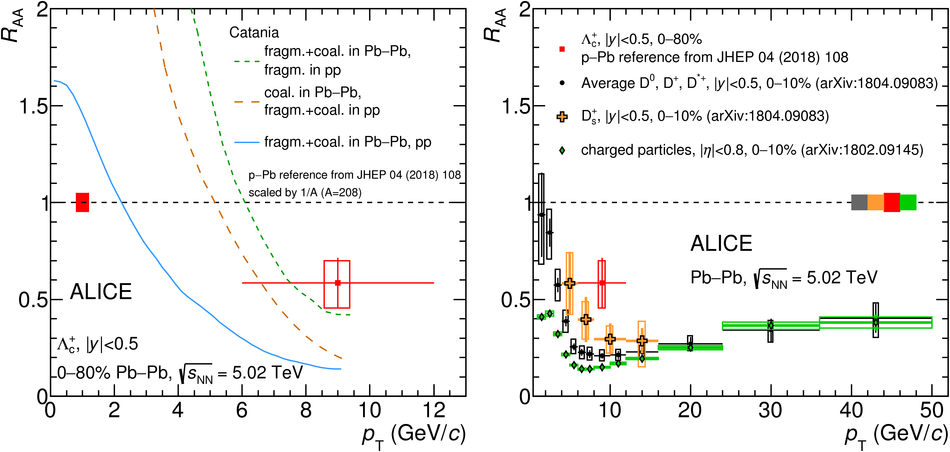# $Λ_\mathrm{c}^+$ production in Pb-Pb collisions at $\sqrt{s_{\rm NN}} = 5.02$ TeV

A measurement of the production of prompt $\Lambda_{\rm c}^{+}$ baryons in Pb-Pb collisions at $\sqrt{s_{\rm NN}} = 5.02$ TeV with the ALICE detector at the LHC is reported. The $\Lambda_{\rm c}^{+}$ and $\overline{\Lambda}_{\rm c}^{-}$ were reconstructed at midrapidity ($|y| <~ 0.5$) via the hadronic decay channel $\Lambda_{\rm c}^{+}\rightarrow {\rm p} {\rm K}_{\rm S}^{0}$ (and charge conjugate) in the transverse momentum and centrality intervals $6 <~ p_{\rm T} <~12$ GeV/$c$ and 0-80%. The $\Lambda_{\rm c}^{+}$/D$^0$ ratio, which is sensitive to the charm quark hadronisation mechanisms in the medium, is measured and found to be larger than the ratio measured in minimum-bias pp collisions at $\sqrt{s} = 7$ TeV and in p-Pb collisions at $\sqrt{s_{\rm NN}} = 5.02$ TeV. In particular, the values in p-Pb and Pb-Pb collisions differ by about two standard deviations of the combined statistical and systematic uncertainties in the common $p_{\rm T}$ interval covered by the measurements in the two collision system. The $\Lambda_{\rm c}^{+}$/D$^0$ ratio is also compared with model calculations including different implementations of charm quark hadronisation. The measured ratio is reproduced by models implementing a pure coalescence scenario, while adding a fragmentation contribution leads to an underestimation. The $\Lambda_{\rm c}^{+}$ nuclear modification factor, $R_{\rm AA}$, is also presented. The measured values of the $R_{\rm AA}$ of $\Lambda_{\rm c}^{+}$, D$_{\rm s}$ and non-strange D mesons are compatible within the combined statistical and systematic uncertainties. They show, however, a hint of a hierarchy $(R_{\rm AA}^{{\rm D}^{0}}<~R_{\rm AA}^{{\rm D}_{\rm s}}<~R_{\rm AA}^{\Lambda_{\rm c}^{+}})$, conceivable with a contribution of recombination mechanisms to charm hadron formation in the medium.

Figures

## Figure 1## Figure 2## Figure 3